# - 8th grade (13y) - math problems

#### Number of problems found: 3354

• TaxesIf you were charged \$243 in taxes on a \$4,050 purchase, what percent tax were you charged?
• Sale goods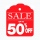Sale goods were reduced twice. First by 15% and then by another 20% of the new price. What percentage of the original price was the final price?
• A diesel carA diesel car costs \$2599 more than an unleaded petrol car and travels an average of 25,000km per year. Diesel petrol has a consumption rate of 7.4L per 100km and costs \$1.39 per litre. Unleaded petrol has a consumption rate of 8.5L per 100km and costs \$1.
• Three 105Three consecutive integers add up to 51. what are these integers?
• The sum 16The sum of A and B is 36. The difference of A & B is 8. find the larger number of A.
• Multiplicative inverse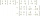Explain why the reciprocal of - 1 1/17 is the multiplicative inverse of - 17/18.
• Two fraction equation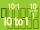Find d value of q in the equation 30/16=q/48.
• Insurance 2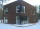A house that is valued at 255,000 euros has been insured for 224,000 euro. At what percent has it been insured?
• A class IV.CIn a class 2/5 were boys. 30 were girls. How many more girls than boys are there?
• Calculate 20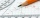Calculate the sum of 1/5 of a right angle and 3/4 of a right angle and 3/4 of a straight angle
• Comparing exponents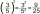A) 8 (6²)(8²) b) 16 (6²)(8²) c) (6¹+²) (8²)
• Over fractionSolve equation: p over 5 equals fraction numerator 7 p over denominator 6 end fraction plus 5
• Regular price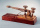The sale price of a television set is 7200. The discount rate is 40%. Find its regular price.
• Taken away from number18 is taken away from 8 times of a number is 30. Find the number.
• The average 4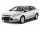The average cost price of a car in Ireland is now €26,430 excluding VAT. If VAT is 23% calculate the actual selling price of a car in 2021.
• The sum 13The sum of the exponents of the prime factors in the prime factorization of 196 is. .. .
• Matilda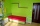Matilda measures the perimeter of her bedroom to determine how many feet of border she needs to go around the tops of the walls. Two walls are each 96 inches long, and two walls are each 3 yards long. She pays \$30 for border that costs \$1 per foot. What a
• Sum and productThe sum of the real numbers x and y is 12. Their difference is 8. What is the value of xy?
• Points OPQ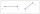Point P is on line segment OQ. Given OP = 6, OQ = 4x - 3, and PQ = 3x, find the numerical length of OQ.
• A trapezoid 3A trapezoid ABCD has the bases length of a = 120 mm, c = 86 mm and the area A = 2,575 mm2. Find the height of the trapezoid.

Do you have an exciting math question or word problem that you can't solve? Ask a question or post a math problem, and we can try to solve it.

We will send a solution to your e-mail address. Solved examples are also published here. Please enter the e-mail correctly and check whether you don't have a full mailbox.

Please do not submit problems from current active competitions such as Mathematical Olympiad, correspondence seminars etc...